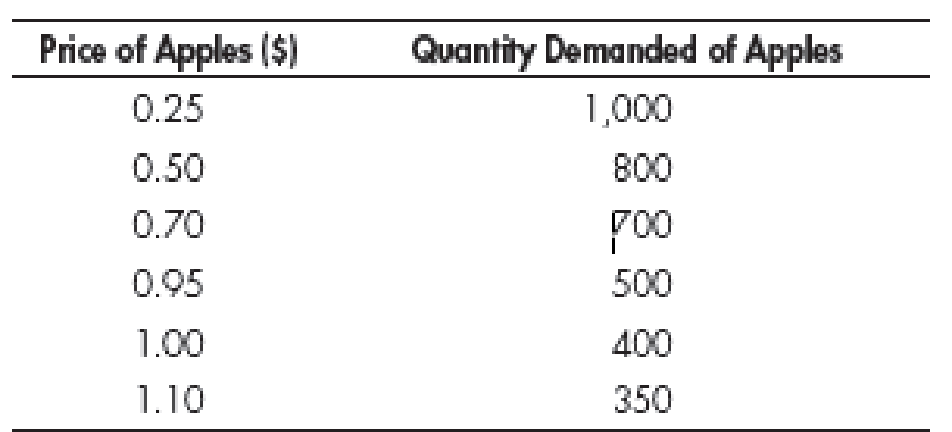Chapter A, Problem 3QP

Chapter
Section
Textbook Problem

Plot the following data, and specify the type of relationship between the two variables. (Place “Price” on the vertical axis and “Quantity Demanded” on the horizontal axis.)To determine

Plot the given data.

Explanation

Table 1 shows the quantity of apple demanded at different price level below:

Table 1

 Price Quantity demanded of apple 0.25 1,000 0.50 800 0.70 700 0.95 500 1.00 400 1.10 350

Figure 1 shows the quantity of apple demanded at different price level below:

Still sussing out bartleby?

Check out a sample textbook solution.

See a sample solution

The Solution to Your Study Problems

Bartleby provides explanations to thousands of textbook problems written by our experts, many with advanced degrees!

Get Started

Chapter A Solutions

Find more solutions based on key concepts